Technical Test SSC JE: Electrical Engineering (EE)- 14

# Technical Test SSC JE: Electrical Engineering (EE)- 14

Test Description

## 100 Questions MCQ Test Mock Test Series for SSC JE Electrical Engineering | Technical Test SSC JE: Electrical Engineering (EE)- 14

Technical Test SSC JE: Electrical Engineering (EE)- 14 for Electrical Engineering (EE) 2022 is part of Mock Test Series for SSC JE Electrical Engineering preparation. The Technical Test SSC JE: Electrical Engineering (EE)- 14 questions and answers have been prepared according to the Electrical Engineering (EE) exam syllabus.The Technical Test SSC JE: Electrical Engineering (EE)- 14 MCQs are made for Electrical Engineering (EE) 2022 Exam. Find important definitions, questions, notes, meanings, examples, exercises, MCQs and online tests for Technical Test SSC JE: Electrical Engineering (EE)- 14 below.
Solutions of Technical Test SSC JE: Electrical Engineering (EE)- 14 questions in English are available as part of our Mock Test Series for SSC JE Electrical Engineering for Electrical Engineering (EE) & Technical Test SSC JE: Electrical Engineering (EE)- 14 solutions in Hindi for Mock Test Series for SSC JE Electrical Engineering course. Download more important topics, notes, lectures and mock test series for Electrical Engineering (EE) Exam by signing up for free. Attempt Technical Test SSC JE: Electrical Engineering (EE)- 14 | 100 questions in 100 minutes | Mock test for Electrical Engineering (EE) preparation | Free important questions MCQ to study Mock Test Series for SSC JE Electrical Engineering for Electrical Engineering (EE) Exam | Download free PDF with solutions
 1 Crore+ students have signed up on EduRev. Have you?
Technical Test SSC JE: Electrical Engineering (EE)- 14 - Question 1

### Resolution of an instrument is

Technical Test SSC JE: Electrical Engineering (EE)- 14 - Question 2

### The volt ampere equation for a long shunt compound motor is given by

Technical Test SSC JE: Electrical Engineering (EE)- 14 - Question 3

### The current through a series RLC circuit under resonance condition will be

Technical Test SSC JE: Electrical Engineering (EE)- 14 - Question 4

Zero-sequence currents can flow from a line to transformer bank if the windings are in

Technical Test SSC JE: Electrical Engineering (EE)- 14 - Question 5

Resistivity of wire depends on

Technical Test SSC JE: Electrical Engineering (EE)- 14 - Question 6

In a transistor largest dimension is that of –

Technical Test SSC JE: Electrical Engineering (EE)- 14 - Question 7

Which one of the following describes correctly the effect of adding a zero to the system ?

Technical Test SSC JE: Electrical Engineering (EE)- 14 - Question 8

For both lap and wave windings, there are as many commutator bars as the number of

Technical Test SSC JE: Electrical Engineering (EE)- 14 - Question 9

A Lissajous pattern on an oscilloscope has 5 horizontal tangencies and 2 vertical tangencies.The frequency of the horizontal input is 1,000 Hz. What is the frequency of the vertical input ?

Technical Test SSC JE: Electrical Engineering (EE)- 14 - Question 10

Negative-sequence reactance of a transformer is

Technical Test SSC JE: Electrical Engineering (EE)- 14 - Question 11

Current flow through a bipolar transistor is by means of –

Technical Test SSC JE: Electrical Engineering (EE)- 14 - Question 12

Five resistances are connected as shown in fig.6.

The equivalent resistance between the point A and B will be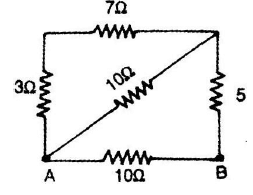Technical Test SSC JE: Electrical Engineering (EE)- 14 - Question 13

The smallest change in a measured variable to which an instrument will respond is

Technical Test SSC JE: Electrical Engineering (EE)- 14 - Question 14

114.Which of the following bridges can be used for inductance measurement ?
1. Maxwell bridge     2. Hay's bridge
3. Wein bridge          4. Wheatstone bridge
Select the correct answer using the code given below:

Technical Test SSC JE: Electrical Engineering (EE)- 14 - Question 15

What is the approximate input impedance of a CRO?

Technical Test SSC JE: Electrical Engineering (EE)- 14 - Question 16

Which of the following relation is incorrect ?

power factor =

Technical Test SSC JE: Electrical Engineering (EE)- 14 - Question 17

Dead time of the instrument is

Technical Test SSC JE: Electrical Engineering (EE)- 14 - Question 18

A FET can be used as a variable –

Technical Test SSC JE: Electrical Engineering (EE)- 14 - Question 19

The power of a three-wire balanced system was measured by two wattmeter method. The reading of one of the wattmeter was found to be double that of the other. What is the pf of the system ?

Technical Test SSC JE: Electrical Engineering (EE)- 14 - Question 20

The field coils of a dc generator are usually made of

Technical Test SSC JE: Electrical Engineering (EE)- 14 - Question 21

Torque/weight ratio of an instrument indicates

Technical Test SSC JE: Electrical Engineering (EE)- 14 - Question 22

......... is the best electronic device for fast switching.

Technical Test SSC JE: Electrical Engineering (EE)- 14 - Question 23

123. The characteristic equation of a feedback control system is given by :s3 + 6s2 + 9s + 4 = 0 is What is the number of roots in the left -half of the s-plane ?

Technical Test SSC JE: Electrical Engineering (EE)- 14 - Question 24

An alternating e.m.f. is applied to a pure inductance such that inductive reactance is 10 W.If the frequency of a.c. is doubled, the reactance will become

Technical Test SSC JE: Electrical Engineering (EE)- 14 - Question 25

A series R-L-C circuit will have unity power factor if operated at a frequency of

Technical Test SSC JE: Electrical Engineering (EE)- 14 - Question 26

The errors introduced by an instrument fall in which category ?

Technical Test SSC JE: Electrical Engineering (EE)- 14 - Question 27

The current drawn by a 120 V dc motor with back emf of 110 V and armature resistance of 0.4W is

Technical Test SSC JE: Electrical Engineering (EE)- 14 - Question 28

If the power factor is below 0.5 in two wattmeter method of power measurement

Technical Test SSC JE: Electrical Engineering (EE)- 14 - Question 29

The positive-negative and zero sequence impedances of a solidly grounded system under steady-state condition always follow the relations

Technical Test SSC JE: Electrical Engineering (EE)- 14 - Question 30

The resistance temperature coefficient is defined as

Technical Test SSC JE: Electrical Engineering (EE)- 14 - Question 31

Which of the following results in a symmetrical fault ?

Technical Test SSC JE: Electrical Engineering (EE)- 14 - Question 32

The advantage of transistor over vaccum tube is –

Technical Test SSC JE: Electrical Engineering (EE)- 14 - Question 33

Accuracy is defined as the

Technical Test SSC JE: Electrical Engineering (EE)- 14 - Question 34

The resistance of the field regulator of a dc shunt motor is of the order of

Technical Test SSC JE: Electrical Engineering (EE)- 14 - Question 35

Which is the pilotless protection method for feeder line ?

Technical Test SSC JE: Electrical Engineering (EE)- 14 - Question 36

A,B,C and D represent the transmission parameters of a two-port network. when is the network reciprocal ?

Technical Test SSC JE: Electrical Engineering (EE)- 14 - Question 37

The condition for the validity under Ohm's law is

Technical Test SSC JE: Electrical Engineering (EE)- 14 - Question 38

The most common type of fault is

Technical Test SSC JE: Electrical Engineering (EE)- 14 - Question 39

The dielectric loss of a capacitor can be measured by which one of the following ?

Technical Test SSC JE: Electrical Engineering (EE)- 14 - Question 40

In Metz-Price percentage differential protection of a D-Y transformer, the CT secondaries connection in the primary and secondary winding of the transformer would be in the form of

Technical Test SSC JE: Electrical Engineering (EE)- 14 - Question 41

Core loss in a dc machine occurs in

Technical Test SSC JE: Electrical Engineering (EE)- 14 - Question 42

The relay used feeder protection is

Technical Test SSC JE: Electrical Engineering (EE)- 14 - Question 43

In a normally biased N-P-N transistor the main current crossing the collectro junction is –

Technical Test SSC JE: Electrical Engineering (EE)- 14 - Question 44

If the power factor is below 0.5 in two wattmeter method of power measurement

Technical Test SSC JE: Electrical Engineering (EE)- 14 - Question 45

Which one of the following bridges is used for measurement of dielectric loss and power factor of a capacitor ?

Technical Test SSC JE: Electrical Engineering (EE)- 14 - Question 46

The load current in short-circuit calculation are neglected because
1. short-circuit current are much larger than load currents
2. short-circuit currents are greatly out of phase with load currents.
Which of these statement (s) is/are correct ?

Technical Test SSC JE: Electrical Engineering (EE)- 14 - Question 47

Which bridge is used to determine frequency ?

Technical Test SSC JE: Electrical Engineering (EE)- 14 - Question 48

The voltage coil of a single phase house energy meter

Technical Test SSC JE: Electrical Engineering (EE)- 14 - Question 49

What should be the main characteristic(s) of the null detector in a bridge measurement ?
1. Accuracy.     2. Precision.
3. Sensitivity.    4. Resolution.
Select the correct answer using the code given below :

Technical Test SSC JE: Electrical Engineering (EE)- 14 - Question 50

Reactance relay is normally used for protection against

Technical Test SSC JE: Electrical Engineering (EE)- 14 - Question 51

The function of circuit breaker is

Technical Test SSC JE: Electrical Engineering (EE)- 14 - Question 52

Holes are drilled on the opposite sides of the disc of an induction type energy meter to

Technical Test SSC JE: Electrical Engineering (EE)- 14 - Question 53

In the potentiometer circuit shown in the given figure, the value of unknown voltage ‘E’ under balanced condition will be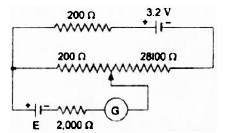Technical Test SSC JE: Electrical Engineering (EE)- 14 - Question 54

The symmetrical components are used in the fault analysis because

Technical Test SSC JE: Electrical Engineering (EE)- 14 - Question 55

Match List I with List II and select the correct answer using the codes given below the lists :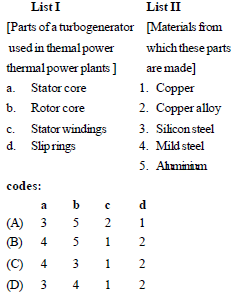Technical Test SSC JE: Electrical Engineering (EE)- 14 - Question 56

In a single-phase induction type energy meter, the lag adjustment is done to ensure that

Technical Test SSC JE: Electrical Engineering (EE)- 14 - Question 57

A capacitor

Technical Test SSC JE: Electrical Engineering (EE)- 14 - Question 58

Consider the following circuit: What is the value of current I in the 5W resistor in the circuit given in the figure ?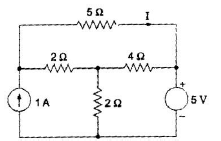Technical Test SSC JE: Electrical Engineering (EE)- 14 - Question 59

What is the nominal pH value of water that is to be maintained in a steam raising thermal power station ?

Technical Test SSC JE: Electrical Engineering (EE)- 14 - Question 60

A cyclindrical rotor synchronous motor is switched on to the supply with its field windings shorted on them selves. It will

Technical Test SSC JE: Electrical Engineering (EE)- 14 - Question 61

What is the characteristic of a good control system ?

Technical Test SSC JE: Electrical Engineering (EE)- 14 - Question 62

A FET is –

Technical Test SSC JE: Electrical Engineering (EE)- 14 - Question 63

The zero-sequence current of a generator for lineto- ground fault is j2.4 pu. Then the current through the neutral during the fault is

Technical Test SSC JE: Electrical Engineering (EE)- 14 - Question 64

Laminations of core are generally made of—

Technical Test SSC JE: Electrical Engineering (EE)- 14 - Question 65

An electric bulb rated 220 V is connected 220 v, 5 Hz source. Then, the bulb

Technical Test SSC JE: Electrical Engineering (EE)- 14 - Question 66

The sensitivity of a Wheatstone bridge depends upon

Technical Test SSC JE: Electrical Engineering (EE)- 14 - Question 67

A fault occurring at the terminals of an unloaded synchronous generator operating at its rate
voltage has resulted in the following values of currents and voltages.

Ia0 = j2.37 pu,    Ia1=j3.05 pu,    Ia2=j0.68 pu,
Va0 = Va1 = Va2 = 0.237 pu

Which one of the following faults has occurred ?

Technical Test SSC JE: Electrical Engineering (EE)- 14 - Question 68

The torque-slip characteristics of a polyphase induction motor becomes almost linear at small values of slips, because in this range of slips,

Technical Test SSC JE: Electrical Engineering (EE)- 14 - Question 69

In which zone the impedance Z is almost pure capacitance ?

Technical Test SSC JE: Electrical Engineering (EE)- 14 - Question 70

The conductor carries more current on the surface in comparison to its core. This phenomenon is called the

Technical Test SSC JE: Electrical Engineering (EE)- 14 - Question 71

In D.C. generator on no-load, the air gap flux distribution in space is—

Technical Test SSC JE: Electrical Engineering (EE)- 14 - Question 72

In a two-Port network, the condition for reciprocity in terms of 'h' - parameters is

Technical Test SSC JE: Electrical Engineering (EE)- 14 - Question 73

In a transmission system, the weight of copper used is proportional to

Technical Test SSC JE: Electrical Engineering (EE)- 14 - Question 74

The speed of a series motor at no load is —

Technical Test SSC JE: Electrical Engineering (EE)- 14 - Question 75

The coupling between two magnetically coupled coils is said to be ideal if the coefficient of coupling  is

Technical Test SSC JE: Electrical Engineering (EE)- 14 - Question 76

A balanced 3-phase system consists of

Technical Test SSC JE: Electrical Engineering (EE)- 14 - Question 77

At which condition of the transformer the equivalent circuit will be as shown in the figure ?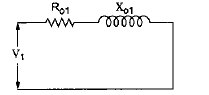Technical Test SSC JE: Electrical Engineering (EE)- 14 - Question 78

In the circuit given in the figure, the limiting error in the power dissipation ‘I2 R’ in the resistor R is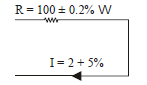Technical Test SSC JE: Electrical Engineering (EE)- 14 - Question 79

In dc machines, the air gap flux distribution in space at no load is

Technical Test SSC JE: Electrical Engineering (EE)- 14 - Question 80

In the ciurcuit shown in the baove figure, the voltage across the 2 ohm resistor is

Technical Test SSC JE: Electrical Engineering (EE)- 14 - Question 81

Linear system obeys

Technical Test SSC JE: Electrical Engineering (EE)- 14 - Question 82

For defining the standard metre, wave length of which material is considered ?

Technical Test SSC JE: Electrical Engineering (EE)- 14 - Question 83

In case of an unbalanced star-connected load supplied from an unbalanced 3-phase, 3-wire
system, load currents will consist of

Technical Test SSC JE: Electrical Engineering (EE)- 14 - Question 84

The permissible flux density in cold rolled grain oriented steel is about

Technical Test SSC JE: Electrical Engineering (EE)- 14 - Question 85

If an R-L circuit having impedance angle f is  awiteched on when figure under sinusoidal voltage wave is passing trough and angle q, there will be no switching transition if

Technical Test SSC JE: Electrical Engineering (EE)- 14 - Question 86

What is the value of the damping ratio of a second order system when the value of the resonant peak is unity ?

Technical Test SSC JE: Electrical Engineering (EE)- 14 - Question 87

Which of the following types of errors come under systematic errors ?
1. Irregular spring tension.
2. Improper readings of an instrument.
4. Error due to the presence of electric field or magnetic field.

Technical Test SSC JE: Electrical Engineering (EE)- 14 - Question 88

The dual of a parallel R-C circuit is a

Technical Test SSC JE: Electrical Engineering (EE)- 14 - Question 89

In a control system , the use of negative feedback

Technical Test SSC JE: Electrical Engineering (EE)- 14 - Question 90

In a power transformer, the breather is provided in order to

Technical Test SSC JE: Electrical Engineering (EE)- 14 - Question 91

Zero-sequence fault current is absent when fault is

Technical Test SSC JE: Electrical Engineering (EE)- 14 - Question 92

A resistance of 105 ohms is specified using significant figures as indicated below :
1. 105 ohms 2. 105.0 ohms
3. 0.000105 MW
Among these

Technical Test SSC JE: Electrical Engineering (EE)- 14 - Question 93

A series motor drawing an armature current of Ia is operating under saturated magnetic conditions. The torque developed in the motor is proportional to

Technical Test SSC JE: Electrical Engineering (EE)- 14 - Question 94

The volume of copper required for an ac transmission line is inversely proportional to

Technical Test SSC JE: Electrical Engineering (EE)- 14 - Question 95

Torque/weight ratio of an instrument indicates

Technical Test SSC JE: Electrical Engineering (EE)- 14 - Question 96

What is the initial slope of Bode magnitude plot of a type-2 system ?

Technical Test SSC JE: Electrical Engineering (EE)- 14 - Question 97

A 3-phase synchronous generator is operating at constant load while the excitation is adjusted to give unity pf current. If the excitation is now increased, the pf will

Technical Test SSC JE: Electrical Engineering (EE)- 14 - Question 98

Which of the following distribution systems is preferred for good efficiency and high economy ?

Technical Test SSC JE: Electrical Engineering (EE)- 14 - Question 99

A combinational circuit

Technical Test SSC JE: Electrical Engineering (EE)- 14 - Question 100

What will be the type of the system, if the steady state performance of control system yields a non zerofinite value of the velocity error constant ?

## Mock Test Series for SSC JE Electrical Engineering

2 videos|1 docs|55 tests
 Use Code STAYHOME200 and get INR 200 additional OFF Use Coupon Code
Information about Technical Test SSC JE: Electrical Engineering (EE)- 14 Page
In this test you can find the Exam questions for Technical Test SSC JE: Electrical Engineering (EE)- 14 solved & explained in the simplest way possible. Besides giving Questions and answers for Technical Test SSC JE: Electrical Engineering (EE)- 14, EduRev gives you an ample number of Online tests for practice

## Mock Test Series for SSC JE Electrical Engineering

2 videos|1 docs|55 tests